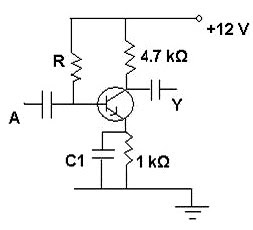## Wednesday, December 01, 2010

### Electronics - Multiple Choice Questions on Transistor Amplifiers

-->
“How strange is the lot of us mortals! Each of us is here for a brief sojourn; for what purpose we know not, though sometimes sense it. But we know from daily life that we exist for other people first of all for whose smiles and well-being our own happiness depends.”
– Albert Einstein
Questions on transistor amplifiers at the class 12 (plus two or higher secondary) level are generally simple and interesting even though some among you may have unclear ideas. Today we will discuss a few questions on common emitter transistor amplifiers:-->
(1) The adjoining figure shows a common emitter transistor amplifier which uses a silicon transistor. If the quiescent emitter current is 1 mA what is the base biasing voltage?
(a) 4.7 V
(b) 3.7 V
(c) 2.7 V
(d) 1.7 V
(e) 0 V
Because of the emitter current the voltage drop across the 1 KΩ resistor connected to the emitter is 1 V.
[1 mA×1 KΩ = (1/1000) A×1000 Ω = 1 V].
The voltage drop across the base-emitter junction of the silicon transistor is 0.7 V. Therefore, the base voltage under no signal (quiescent) condition is 1 V + 0.7 V = 1.7 V.
(2) In the amplifier circuit shown in Question No.1 what is the function of the capacitor C1 connected across the 1 KΩ emitter resistor?
(a) To produce positive feed back.
(b) To produce negative feed back.
(c) To pass the excess signal to the ground.
(d) To act as filter capacitor for the transistor supply voltage.
(e) To bypass the signal current so that it will not flow through the emitter resistor.
The capacitor C1 provides an easy path (bypass) for the signal component of the emitter current. If C1 is absent the signal component of the emitter current will produce signal voltage drop across the emitter resistor, thereby reducing the signal output at the collector.
The correct option is (e).
(3) If the common emitter current gain βdc of the transistor used in the amplifier circuit shown in Question No.1 is 200, the quiescent base current of the transistor is very nearly equal to
(a) 1 mA
(b) 1 μA
(c) 2 μA
(d) 4 μA
(e) 5 μA
In the common emitter mode, the current amplification factor (current gain) under no signal condition (βdc) is given by
βdc = IC/IB where IC is the collector current and IB is the base current (both under no signal conditions).
Since the collector current is almost equal to the emitter current IE (because of large value of βdc), we have
βdc ≈ IE/IB
Therefore IB ≈ IE/βdc = 1 mA/200 = 0.005 mA = 5 μA.
(4) If the common emitter current gain βdc of the transistor used in the amplifier circuit shown in Question No.1 is 200, what is the voltage drop across the base biasing resistor R under quiescent conditions?
(a) 12 V
(b) 11 V
(c) 10.3 V
(d) 5.4 V
(e) 4.7 V
The quiescent base current is 5 μA as shown in answering question no.3 above. The base biasing voltage is 1.7 V as shown in answering question no.1. The power supply voltage is 12 V. Therefore, the voltage drop across the base biasing resistor R under quiescent conditions is 12 V – 1.7 V = 10.3 V.
(5) The base biasing resistor in the circuit shown in Question No.1 is
(a) 1 KΩ
(b) 4.7 KΩ
(c) 1.03 MΩ
(d) 1.87 MΩ
(e) 2.06 MΩ
The quiescent base current of the transistor is very nearly equal to 5 μA as shown in answering Question No.3. The voltage drop across the base biasing resistor R under quiescent conditions is 10.3 V as shown in answering Question No.4. Therefore, the base biasing resistor is given by
R = (10.3)V/(5 μA) = 2.06 MΩ
-->
You can access all questions (with solution) on electronics posted on this site by clicking on the label ‘electronics’ below this post or by trying a search for ‘electronics’ using the search box provided on this page
-->